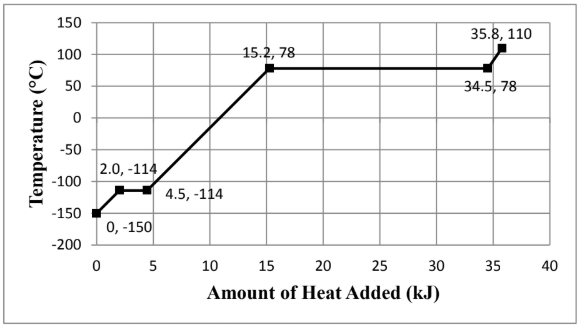# Problem: Consider the heating curve shown below for 22.86 grams of an unknown substance. You may assume constant pressure conditions. Note: the points are labeled as (amount of heat added, temperature). The identity of the unknown substance whose heating curve is shown above is...(Circle your final answer). The moles were identified to be 0.496 mol.A) Ethane, C2H6. B) Ethanol, C2H6O. C) Propene, C3H6. D) Butanol, C4H10O.Show all work:

###### FREE Expert Solution

We are asked to get the identity of the unknown substance whose heating curve is shown above.

The molar mass can help us identify the identity of the substance.

We go through the following steps to solve this problem:

Step 1. Calculate the molar mass of the substance

Step 2. Calculate the molar mass of the options

Step 3. Identify the compound

98% (310 ratings)###### Problem Details

Consider the heating curve shown below for 22.86 grams of an unknown substance. You may assume constant pressure conditions. Note: the points are labeled as (amount of heat added, temperature).The identity of the unknown substance whose heating curve is shown above is...(Circle your final answer). The moles were identified to be 0.496 mol.

A) Ethane, C2H6

B) Ethanol, C2H6O.

C) Propene, C3H6

D) Butanol, C4H10O.

Show all work: